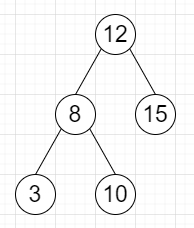# Program to find number of nodes in a range in Python

Suppose we have a BST, and we also have left and right bounds l and r, we have to find the count of all nodes in root whose values are present in between l and r (inclusive).

So, if the input is likel = 7, r = 13, then the output will be 3, as there are three nodes: 8, 10, 12.

To solve this, we will follow these steps−

• stack := a stack and insert root at first, count := 0

• while stack is not empty, do

• node := top element of stack, and pop element

• if node is not null, then

• if l <= data of node <= r, then

• count := count + 1

• stack := push right of node and left of node into stack

• otherwise when data of node < l, then

• stack := push right of node into stack

• otherwise,

• stack := push left of node into stack

• return count

## Example

Live Demo

from collections import deque
class TreeNode:
def __init__(self, data, left = None, right = None):
self.data = data
self.left = left
self.right = right
class Solution:
def solve(self, root, l, r):
stack, count = [root], 0
while stack:
node = stack.pop()
if node:
if l <= node.data <= r:
count += 1
stack += [node.right, node.left]
elif node.data < l:
stack += [node.right]
else: stack += [node.left]
return count
ob = Solution()
root = TreeNode(12)
root.left = TreeNode(8)
root.right = TreeNode(15)
root.left.left = TreeNode(3)
root.left.right = TreeNode(10)
print(ob.solve(root, 7,13))



## Input

root = TreeNode(12)
root.left = TreeNode(8)
root.right = TreeNode(15)
root.left.left = TreeNode(3)
root.left.right = TreeNode(10)
7,13

## Output

3